shell运算符_shell运算符：shell算数运算符、关系运算符、布尔运算符、字符串运算符等 - CSDN

• Bash 支持很多运算符，其中包括算数运算符、关系运算符、布尔运算符、字符串运算符和文件测试运算符
目录
文章目录目录概述1. 算数运算符2. 关系（比较）运算符3. 逻辑运算符4. 字符串运算符5. 文件测试运算符
概述
Bash 支持很多运算符，其中包括：
 - 算数运算符
- 关系（比较）运算符
- 逻辑运算符
- 字符串运算符
- 文件测试运算符

1. 算数运算符
a=10
b=20


算术运算符
说明
举例

+
加法
expr $a +$b 结果为30

-
减法
expr $a -$b 结果为-10

*
乘法
expr $a \*$b 结果为200

/
除法
expr $b /$2 结果为2

%
取余
expr $b %$a 结果为0

=
赋值
a=$b 把变量b的值赋值给变量a == 相等。判断两个数字是否相等，相等则返回true [$a == $b ] 返回true != 不相等。判断两个数字是否相等，不相等则返回true [$a != $b ] 返回true 其实原生bash不支持简单的数学运算，但是可以通过其他命令来实现， 例如: awk 和 expr命令进行处理计算之后才可以得出结果，expr 最常用。 expr 是一款表达式计算工具，使用它能完成表达式的求值操作。 # 以下几点需要注意： 1.表达式和运算符之间要有空格。 例如： 5+10 是不对的，必须写成 5 + 10，这与我们熟悉的大多数编程语言不一样。 2.完整的表达式要被   包含，注意这个字符不是常用的单引号，在 Esc 键下边。 3.乘号(*)前边必须加反斜杠(\)进行转义才能实现乘法运算。  举例说明： #!/bin/bash #created by yuki on 20180723 #this script is used for doing ...... a=10 b=20 result=expr$a + $b echo "a + b =$result"

result=expr $a -$b
echo "a - b = $result" result=expr$a \* $b echo "a * b =$result"

result=expr $b /$a
echo " b / a = $result" result=expr$b % $a echo "b % a =$result"

运行结果：
a + b = 30
a - b = -10
a * b = 200
b / a = 2
b % a = 0

2. 关系（比较）运算符
a=5
b=10

关系运算符包括：

在[]中使用的比较符
在(()和[[]]}中使用的比较符
说明
举例

-eq
==
equal的缩写，表相等
[ $a -eq$b ] 返回 false

-ne
!=
not equal的缩写，表不相等
[ $a -nq$b ] 返回 true

-gt
>
greater thanl的缩写，表大于
[ $a -gt$b ] 返回 false

-ge
>=
greater equal的缩写，表大于等于
[ $a -ge$b ] 返回 false

-lt
<
greater equal的缩写，表小于
[ $a -lt$b ] 返回 true

-le
<=
greater equal的缩写，表小于等于
[ $a -lq$b ] 返回 true

提示：
1. "<"符号意思是小于，要麽：if [[ "$a" < "$b" ]] 是错误的，要麽：if [ "$a" \< "$b" ] （即在单[]中需要转义，因为shell也用 <和>表示重定向）。
2. ">"符号意思是大于，要麽：if [[ "$a" > "$b" ]] 是错误的，要麽：if [ "$a" \> "$b" ] （即在单[]中需要转义，因为shell也用 <和>表示重定向）。
3. 关系运算符只支持数字（整数），不支持字符串，除非字符串的值是数字（整数）。
4. 条件表达式要放在方括号之间，并且要有空格，例如 [[$a==$b]] 是错误的，必须写成 [[ $a ==$b ]]。

举例说明：
#!/bin/bash

#created by yuki on 20180723
#this script is used for doing ......

a=5
b=10

if [ $a -eq$b ]
then
echo "eq 关系运算结果: a等于b"
else
echo "eq 关系运算结果: a不等于b"
fi

if [ $a -ne$b ]
then
echo "ne 关系运算结果: a不等于b"
else
echo "ne 关系运算结果: a等于b"
fi

if [ $a -gt$b ]
then
echo "gt 关系运算结果: a大于b"
else
echo "gt 关系运算结果: a小于等于b"
fi

if [ $a -lt$b ]
then
echo "lt 关系运算结果：a小于b"
else
echo "lt 关系运算结果：a大于等于b"
fi

if [ $a -ge$b ]
then
echo "ge 关系运算结果：a大于等于b"
else
echo "ge 关系运算结果：a小于b"
fi

if [ $a -le$b ]
then
echo "le 关系运算结果：a小于等于b"
else
echo "le 关系运算结果：a大于b"
fi

运行结果：
eq 关系运算结果: a不等于b
ne 关系运算结果: a不等于b
gt 关系运算结果: a小于等于b
lt 关系运算结果：a小于b
ge 关系运算结果：a小于b
le 关系运算结果：a小于等于b

3. 逻辑运算符

在[ ]中使用的比较符
在(()和[[]]}中使用的比较符
说明
举例

赋值
a=10
b=25

-a
&&
与运算，两个表达式都为true,才返回true
[ $a -lt 20 -a$b -gt 20 ] 返回true

-o
||
或运算，有一个表达式都为true,则返回true
[ $a -lt 20 -o$b -gt 100 ] 返回true

!
!
非运算，表达式为true,则返回false
[ ! false ] 返回true

小结：逻辑操作符使用总结
[ ]中用 -a,-o, !
[[ ]]中用 &&,||, !
test 用法和 [ ]相同

举例说明：
#!/bin/bash

#created by yuki on 20180723
#this script is used for doing ......

a=5
b=10

if [ $a !=$b ]
then
echo "!= 逻辑非运算结果：a不等于b"
else
echo "!=逻辑非运算结果： a等于b"
fi

if [ $a -lt 50 -a$b -gt 8 ]
then
echo "and 逻辑与运算结果：真"
else
echo "and 逻辑与运算结果：假"
fi

if [ $a -lt 50 -o$b -gt 12 ]
then
echo "or 逻辑或运算结果：真"
else
echo "or 逻辑或运算结果：假"
fi

if [ $a -lt 3 -o$b -gt 8 ]
then
echo "or 逻辑或运算结果：真"
else
echo "or 逻辑或运算结果：假"
fi

运行结果：
!= 逻辑非运算结果：a不等于b
and 逻辑与运算结果：真
or 逻辑或运算结果：真
or 逻辑或运算结果：真

4. 字符串运算符
字符串运算符包括：

字符串运算符
说明
举例

=
检测两个字符串是否相等，相等则返回true
[ $a =$b ] 返回true

!=
检测两个字符串是否相等，不相等则返回true
[ $a !=$b ] 返回true

-z
检测字符串长度是否为0，为0则返回true
[ -z $b ] 返回true -n 检测字符串长度是否为0，不为0则返回true [ -n$b ] 返回true

str
检测字符串是否为null，不为null则返回true
[  $b ] 返回true #经测试： 字符串比较用单等号或双等号都可以，而且外边的中括号最好是用双中括号，这样结果比较准确。  举例说明： [root@myhost ~]# string="abcde" [root@myhost ~]# if [[ "$string" == abc* ]]; then  echo "abc开头"; else echo "不是"; fi
abc开头
[root@myhost ~]# if [ "$string" == abc* ]; then echo "abc开头"; else echo "不是"; fi 不是 [root@myhost ~]# if [ "$string" = abc* ]; then  echo "abc开头"; else echo "不是"; fi
不是
[root@myhost ~]# if [[ "$string" = abc* ]]; then echo "abc开头"; else echo "不是"; fi abc开头 [root@myhost ~]# string="abcde" [root@myhost ~]# if [[ "$string" = "abc*" ]]; then  echo "不相等"; else echo "不等"; fi
不等
[root@myhost ~]# if [[ "$string" == "abc*" ]]; then echo "不相等"; else echo "不等"; fi 不等 [root@myhost ~]# if [ "$string" == "abc*" ]; then  echo "不相等"; else echo "不等"; fi
不等
[root@myhost ~]# if [ "$string" = "abc*" ]; then echo "不相等"; else echo "不等"; fi 不等  举例说明：  #!/bin/bash #created by yuki on 20180723 #this script is used for doing ...... a="cfe" b="efr" if [ "$a" = "$b" ] then echo "字符串a和字符串b相等" else echo "字符串a和字符串b不相等" fi if [ "$a" == "$b" ] then echo "字符串a和字符串b相等" else echo "字符串a和字符串b不相等" fi [root@myhost ~]# a="cfe" [root@myhost ~]# b="efr" [root@myhost ~]# if [ "$a" =="$b" ] > then ############## end #################################### if [ "$a" != "$b" ] then echo "字符串a和字符串b不相等" else echo "字符串a和字符串b相等" fi if [ -z "$a" ]
then
echo "字符串a的长度为0,即字符串a为空？"
else
echo "字符串a的长度不为0"
fi

if [ -n "$a" ] then echo "字符串a的长度不为0" else echo "字符串a的长度为0,即字符串a为空？" fi if [ "$a" ]
then
echo "字符串a不为空"
else
echo "字符串不为空"
fi

运行结果：
字符串a和字符串b不相等
字符串a的长度不为0
字符串a的长度不为0
字符串a不为空

5. 文件测试运算符
文件测试运算符包括：

文件测试运算符
说明
举例

-b
检测文件是否是块设备文件，如果是，则返回true
[ -b $file ] 返回true -c 检测文件是否是字符设备文件，如果是，则返回true [ -c$file ] 返回true

-d
检测文件是否是目录文件，如果是，则返回true
[ -d $file ] 返回true -f 检测文件是否是普通文件（既不是目录也不是设备文件），如果是，则返回true [ -f$file ] 返回true

-g
检测文件是否设置了SGID位，如果是，则返回true
[ -g $file ] 返回true -k 检测文件是否设置了粘着位（stucky Bit），如果是，则返回true [ -k$file ] 返回true

-p
检测文件是否具名管道，如果是，则返回true
[ -p $file ] 返回true -u 检测文件是否设置了SUID位，如果是，则返回true [ -u$file ] 返回true

-r
检测文件是否可读，如果是，则返回true
[ -r $file ] 返回true -w 检测文件是否可写，如果是，则返回true [ -w$file ] 返回true

-x
检测文件是否可执行，如果是，则返回true
[ -x $file ] 返回true -s 检测文件是否为不为空（文件大小是否不为0），如果不为0，则返回true [ -s$file ] 返回true

-e
检测文件(包括目录)是否存在，如果存在，则返回true
[ -e $file ] 返回true -a 检测文件(包括目录)是否存在，如果存在，则返回true [ -e$file ] 返回true

文件测试运算符用于检测 Unix 文件的各种属性，-a已经被弃用了。

举例说明：
例如，变量 file 表示文件“ /etc/rc.d/rc.local”，它的大小为220kb，所有者root用户具有 rwx 权限。
[root@myhost ~]# ll /etc/rc.d/rc.local
-rwxr-xr-x 1 root root 220 Oct  4  2017 /etc/rc.d/rc.local

下面的代码，将检测该文件的各种属性：
#!/bin/bash

#created by yuki on 20180723
#this script is used for doing ......

file="/etc/rc.d/rc.local"

if [ -e $file ] then echo "这个已经存在" else echo "这个不存在" fi if [ -r$file ]
then
echo "当前用户可读此文件"
else
echo "当前用户没有读此文件的权限"
fi

if [ -w $file ] then echo "当前用户可写此文件" else echo "当前用户没有写此文件的权限" fi if [ -x$file ]
then
echo "当前用户可以执行此文件"
else
echo "当前用户没有执行此文件的权限"
fi

if [ -d $file ] then echo "这个是目录" else echo "这个不是目录" fi if [ -f$file ]
then
echo "这个是普通文件"
else
echo "这个不是普通文件"
fi

if [ -s $file ] then echo "此文件大小不为0" else echo "此文件大小为0,即文件内容为空" fi  运行结果： 这个已经存在 当前用户可读此文件 当前用户可写此文件 当前用户可以执行此文件 这个不是目录 这个是普通文件 此文件大小不为0   展开全文• Shell 基本运算符Shell 和其他编程语言一样，支持多种运算符，包括：算数运算符关系运算符布尔运算符字符串运算符文件测试运算符原生bash不支持简单的数学运算，但是可以通过其他命令来实现，例如 awk 和 expr，expr... Shell 基本运算符Shell 和其他编程语言一样，支持多种运算符，包括：算数运算符关系运算符布尔运算符字符串运算符文件测试运算符原生bash不支持简单的数学运算，但是可以通过其他命令来实现，例如 awk 和 expr，expr 最常用。expr 是一款表达式计算工具，使用它能完成表达式的求值操作。例如，两个数相加(注意使用的是反引号  而不是单引号 ')：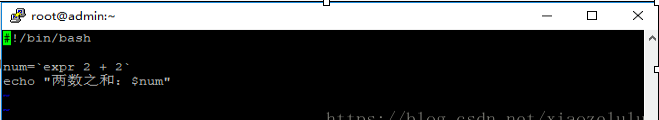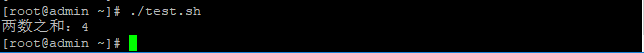两点注意：表达式和运算符之间要有空格，例如 2+2 是不对的，必须写成 2 + 2，这与我们熟悉的大多数编程语言不一样。完整的表达式要被   包含，注意这个字符不是常用的单引号，在 Esc 键下边。算术运算符下表列出了常用的算术符，假定变量 a 为 10，变量 b 为 20：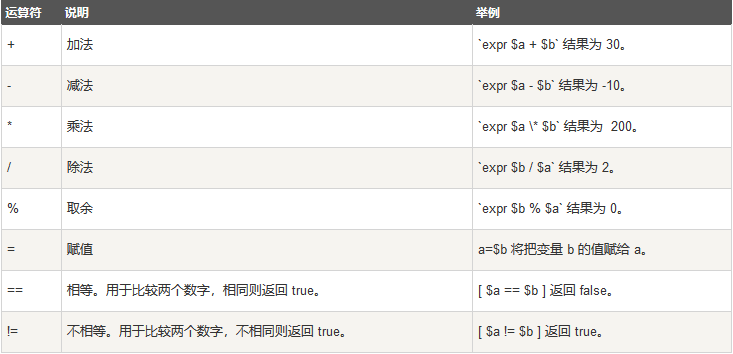注意：条件表达式要放在方括号之间，例子： a=1 b=2 echo$[$a +$b ]     echo expr $a +$b结果：    3               3       ① 将进行运算的表达式的的结果[ ],得到一个值。       ② 表达式的结果需要用expr，和反单引号。关系运算符关系运算符只支持数字，不支持字符串，除非字符串的值是数字。下表列出了常用的关系运算符，假定变量 a 为 10，变量 b 为 20：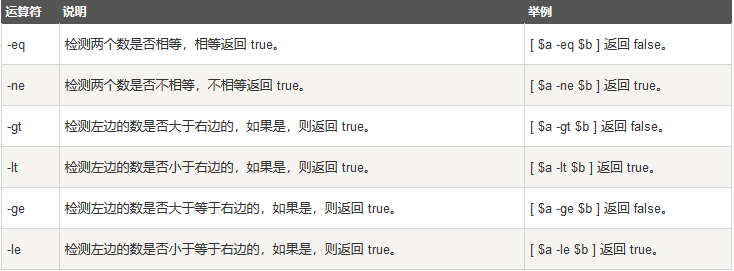if [ $a -eq$b ]
then
echo "$a -eq$b : a 等于 b"
else
echo "$a -eq$b: a 不等于 b"
fi布尔运算符 下表列出了常用的布尔运算符，假定变量 a 为 10，变量 b 为 20：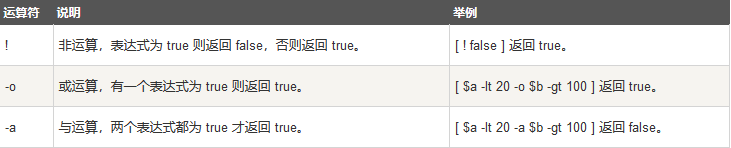if [ $a !=$b ]
then
echo "$a !=$b : a 不等于 b"
else
echo "$a !=$b: a 等于 b"
fi逻辑运算符以下介绍 Shell 的逻辑运算符，假定变量 a 为 10，变量 b 为 20:字符串运算符下表列出了常用的字符串运算符，假定变量 a 为 "abc"，变量 b 为 "efg"：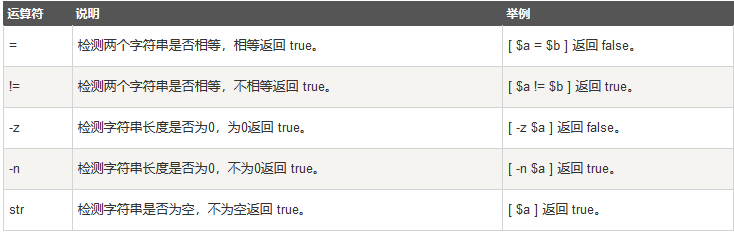echo $[$a = $b]不相等，输出0；文件测试运算符文件测试运算符用于检测 Unix 文件的各种属性。属性检测描述如下：file="/var/www/runoob/test.sh" if [ -r$file ]
then
echo "文件可读"
else
echo "文件不可读"
fi

结果：文件可读

展开全文• 变量本质上存储数据的一个或多个计算机内存地址，分为本地变量（用户当前shell生命期使用，随shell进程的消亡而无效，类似局部变量）、环境变量（适用于所有由登录进程所产生的子进程）和位置参数（向shell脚本传递...

一、简介
Shell各种判断结构和运算符的用法是shell编程的基础，了解shell的判断、运算符和一些退出状态对后面的学习有很重要的影响。shell有一个内部命令test经常用于对判断语句进行测试一种或几种状态的条件是否成立。

二、详解
1、测试和运算符
（1）Linux的shell中的测试命令，用于测试某种条件或某几种条件是否真实存在。测试命令是判断语句和循环语句中条件测试的工具，对判断和运算符的比较测试有很大的帮助。
（2）测试条件为真，返回一个0值；为假，返回一个非0整数值。测试命令有两种方式，一种test expression（表达式）；另一种命令格式[ expression ]，其中"["是启动测试命令，"]"要与之配对，而且"["和"]"前后的空格必不可少，此方式常作为流程控制语句的判断条件。
（3）整数比较运算符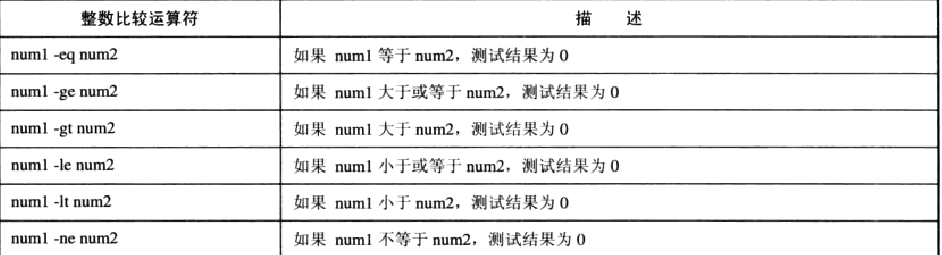格式：test "num1" operator "num2"或[ "num1" operator "num2" ]，测试结果为真用0表示，为假用非0表示。但只能用于比较数值的大小， 不可用于字符串、文件操作（字符串比较运算符和文件操作符也不能用于其他的操作）。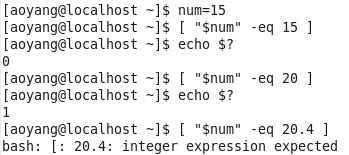对浮点型数值进行比较，不能使用整型运算符。
（4）字符串运算符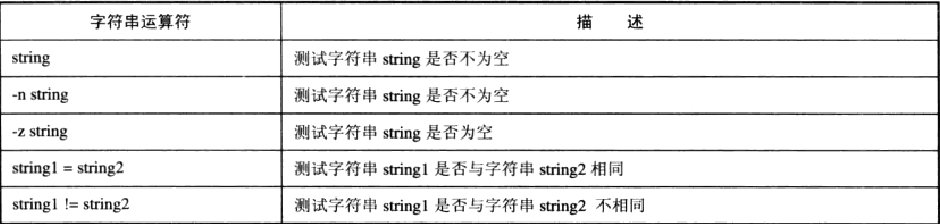Shell编程是严格区分大小写的，并注意空格的问题，运算符左右的空格不能少。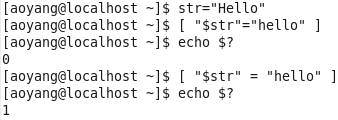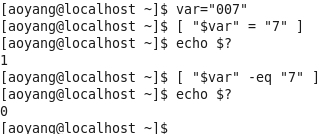字符串赋值和整数赋值没有区别，而shell对变量弱化了，因此不要把字符串比较运算符当作整数比较运算符使用。
（5）文件操作符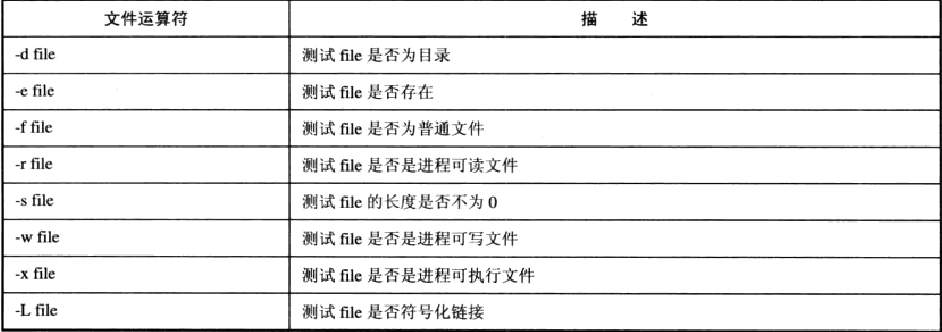文件操作符中的可读、可写、可执行的权限判断经常和chmod命令联用。
（6）逻辑运算符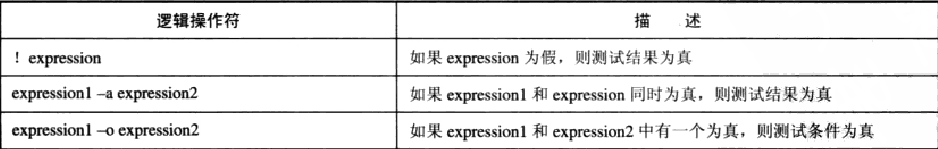用于测试多个条件是否为真或为假，也可使用逻辑非测试单个表达式。
表达式：expression1 -a expression1 -aexpression1（并不是所有的运算符都会被执行，只有表达式expression1为真，才会测试expression2为真。只有expression1和expression2都为真才会接着测试expression3是否为真。）
表达式：expression1 -aexpression1 -aexpression1（只要expression1为真，就不用去测试表达式expression2和expression3。只有expression1为假时才会去判断表达式expression2和expression3。同样，只有expression2和expression3同时为假时才会去测试expression3）。
例如：判断文件存在并cd切换目录，[-e /tmp/test -a -d /tmp/test ] || cd /tmp/test或[-e /tmp/test ] && [ -d /tmp/test ] || cd /tmp/test
例如：文件可执行则启动，[-x /etc/init.d/network] && result=/etc/init.d/network start
（7）算术运算符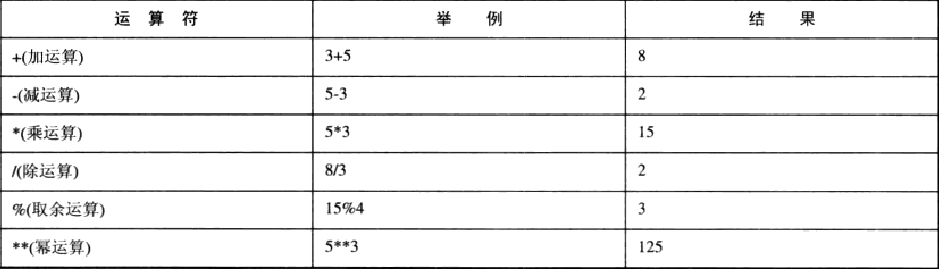使用let命令来执行算术运算，除法和取余运算过程中要注意除数不能为0，使用算术运算符无法对字符串、文件、浮点型数进行计算（浮点型操作，需要用到专门的函数）。
算术运算符与赋值运算符"="联用，称为算术复合赋值运算符。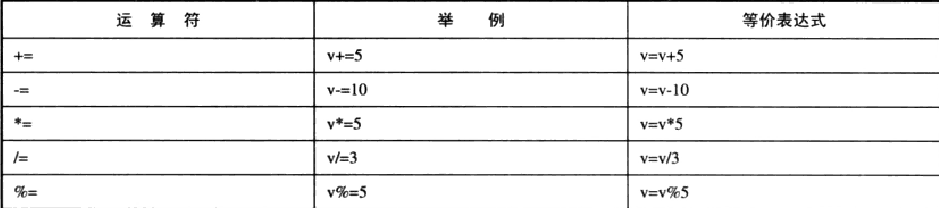（8）位运算符
用于整数间的运算，按位与运算只有两个二进制都为1，结果才为1；按位或运算只要有一个二进制位为1，则结果为1；按位异或运算两个二进制位数相同时，结果为0，否则为1。按位取反运算符将二进制中的0修改成1，1修改成0。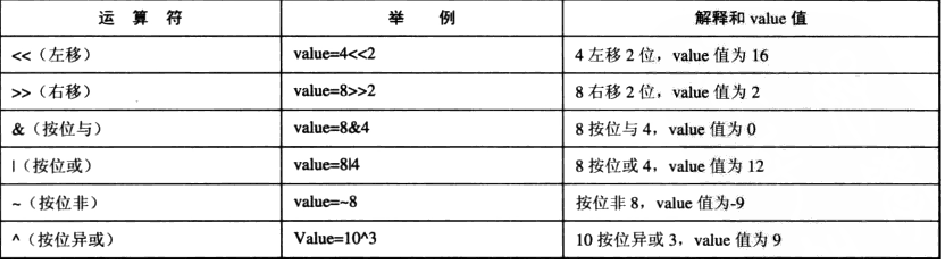位运算符同样可以同赋值运算符联用，组成复合赋值运算符。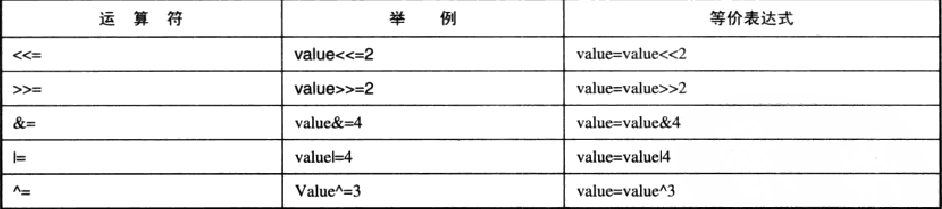（9）自增自减运算符
自增自减运算符包括前置自增、前置自减、后置自增和后置自减。自增自减操作符的操作元只能是变量，不能是常数或表达式，且该变量值必须为整数型。

#!/bin/sh

num1=5

let "a=5+(++num1) "
echo "a=$a" num2=5 let "b=5+(num2++) " echo "b=$b"（10）数字常量

Shell脚本或命令默认将数字以十进制的方式进行处理，当使用0作为前缀时表示八进制，当使用0x进行标记时表示十六进制，同时还可使用num#这种形式标记进制数。

#!/bin/sh

let "num1=40"
echo "num1=$num1" let "num2=040" echo "num2=$num2"

let "num3=0x40"
echo "num3=$num3"分别使用设置数字常量40的十进制、八进制和十六进制的表示方式，产生的十进制结果是不同的。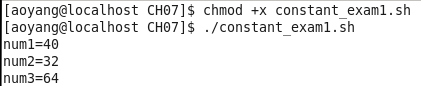#!/bin/sh let "num1=2#1101100110001101" echo "num1=$num1"

let "num2=8#50"
echo "num2=$num2" let "num3=16#50" echo "num3=$num3"2、Bash数学运算
（1）expr命令
expr一般用于整数值计算和字符串的操作。其操作符名称如下表所示。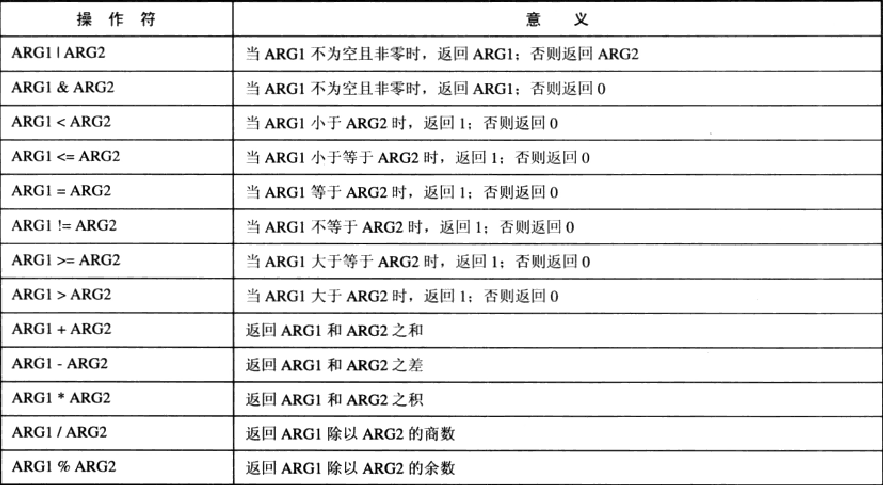注：若expr的操作符是元字符（不是普通字符），需要用转义符将操作符的特殊含义屏蔽，进行数学运算，如expr 2014 \* 2。expr操作符的两端必须有空格，否则不会执行数学运算expr 2014 - 2008。
（2）bc运算器
bc是一种内建的运算器，是bash shell中最常用的浮点数运算工具，包括整型数和浮点数、数组变量、表达式、复杂程序结构和函数。
bc运算器支持的数学运算符号如下表：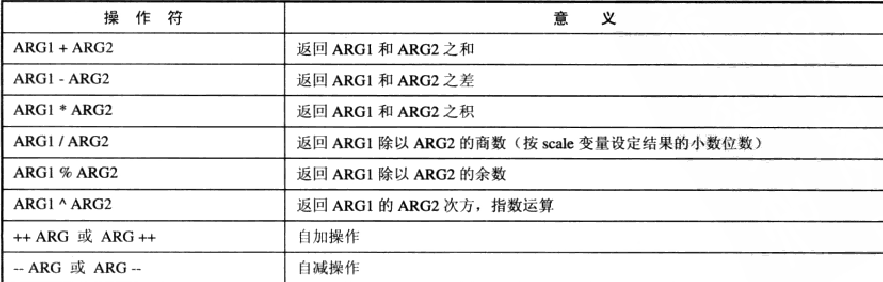bc运算器定义了内建变量scale用于设定除法运算的精度（默认scale=0）。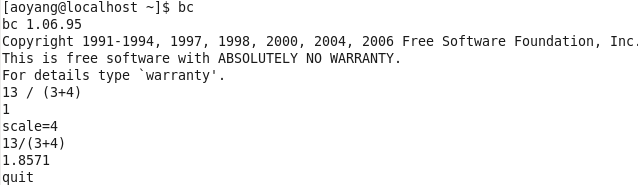scale设为4后，除法结果小数点后保留4位。bc -q可以使bc运算器不输出版本信息。
在shell中用bc运算器进行浮点数运算需要使用命令替换的方式。脚本中调用bc运算器一般格式：variable=echo "options; expression" | bc。

#!/bin/bash

var1=20
var2=3.14159
var3=echo "scale=5; $var1 ^ 2" | bc var4=echo "scale=5;$var3 * $var2" | bc echo "The area of this circle is:$var4"bc运算器的指数运算计算var1变量的平方，scale=5输出结果的小数点后精确到第5位。

3、退出状态

（1）Linux系统，每当命令执行完成后，系统返回一个退出状态。若退出状态值为0，表示命令运行成功；反之若退出状态值不为0，则表示命令运行失败。最后一次执行命令的退出状态值被保存在内置变量"$?"中。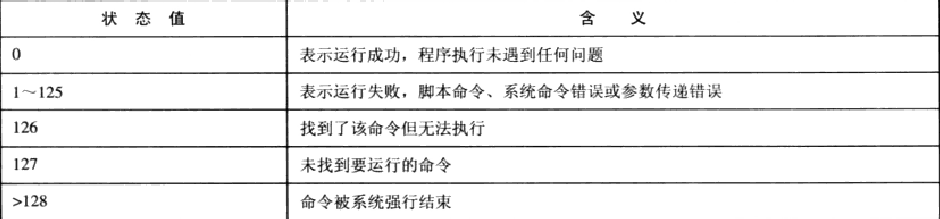（2）exit命令格式：exit status（status在0～255之间），返回该状态值时伴随脚本的退出，参数被保存在shell变量$?中。

3、IF判断

（1）if、then、else语句用于判断给定的条件是否满足，并根据测试条件的真假来选择相应的操作。if/else仅仅用于两分支判断，多分支的选择时需要用到if/else语句嵌套、if/elif/else和case多分支选择判断结构。
（2）if结构

#!/bin/sh

if [ "$integer1" -lt 15 ] then echo "The integer which you input is lower than 15." fi 注意：测试条件后如果没有";"则then语句要换行。 （3）if/else结构 #!/bin/sh echo "Please input the file which you want to delete:" read file if rm -f "$file"
then
echo "Delete the file $file sucessfully!" else echo "Delete the file$file failed!"
fi（4）if/else语句嵌套

可同时判断三个或三个以上条件，但要注意if与else配对关系，else语句总是与它上面最近的未配对的if配对。

#!/bin/bash
#提示用户输入分数（0-100）
echo "Please Input a integer(0-100): "

#判断学生的分数类别
if [ "$score" -lt 0 -o "$score" -gt 100 ]
then
echo "The score what you input is not integer or the score is not in (0-100)."
else
if [ "$score" -ge 90 ] then echo "The grade is A!" else if [ "$score" -ge 80 ]
then
else
if [ "$score" -ge 70 ] then echo "The grade is C!" else if [ "$score" -ge 60 ]
then
else
fi
fi
fi
fi
fi（4）if/elif/else结构

if/else嵌套在编程中很容易漏掉then或fi产生错误，而且可读性很差，因此引入if/elif/else结构针对某一事件的多种情况进行处理，fi只出现一次，可读性也提高了。
#!/bin/bash

echo "Please Input a integer(0-100): "

if [ "$score" -lt 0 -o "$score" -gt 100 ]
then
echo "The score what you input is not integer or the score is not in (0-100)."
elif [ "$score" -ge 90 ] then echo "The grade is A!" elif [ "$score" -ge 80 ]
then
elif [ "$score" -ge 70 ] then echo "The grade is C!" elif [ "$score" -ge 60 ]
then
else
fi例：判断输入的年份是否是润年（润年条件：1、能被4整除，但不能被100整除的年份。2、能被100整除，又能被400整除的年份）

#!/bin/sh

echo "Please Input a year: "

let "n1=$year % 4" let "n2=$year % 100"
let "n3=$year % 400" if [ "$n1" -ne 0 ]
then
leap=0
elif [ "$n2" -ne 0 ] then leap=1 elif [ "$n3" -ne 0 ]
then
leap=0
else
leap=1
fi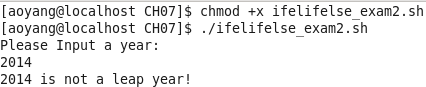（5）case结构
case结构变量值依次比较，遇到双分号则跳到esac后的语句执行，没有匹配则脚本将执行默认值"*)"后的命令，直到"';;"为止。case的匹配值必须是常量或正则表达式。

#!/bin/bash

echo "Please Input a score_type(A-E): "

case "$score_type" in A) echo "The range of score is from 90 to 100 !";; B) echo "The range of score is from 80 to 89 !";; C) echo "The range of score is from 70 to 79 !";; D) echo "The range of score is from 60 to 69 !";; E) echo "The range of score is from 0 to 59 !";; *) echo "What you input is wrong !";; esac 三、总结 （1）shell的各种运算符是编程的重要环节。IF和CASE条件判断结构的应用，使问题有了多分支的选择。 （2）本文仅简单的介绍重要的基础知识，若要熟练使用，还需要大量的练习。  展开全文• Shell运算符的分类 一，算数运算符 expr是一款表达式计算工具，使用它就能完成表达式的求值操作。 ①两个数相加,使用的是反引号 ，而不是单引号,在左上角的Esc键下方 ②expr后面的数字和符号都要空格（例如...  Linux中的Shell基本运算符 Shell运算符的分类一，算数运算符 expr是一款表达式计算工具，使用它就能完成表达式的求值操作。 ①两个数相加,使用的是反引号 ，而不是单引号,在左上角的Esc键下方 ②expr后面的数字和符号都要空格（例如num=expr 1 + 2）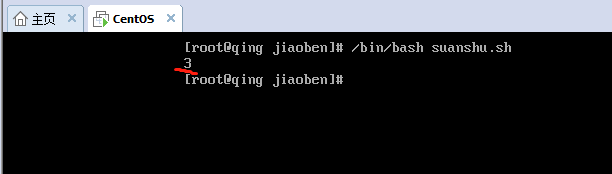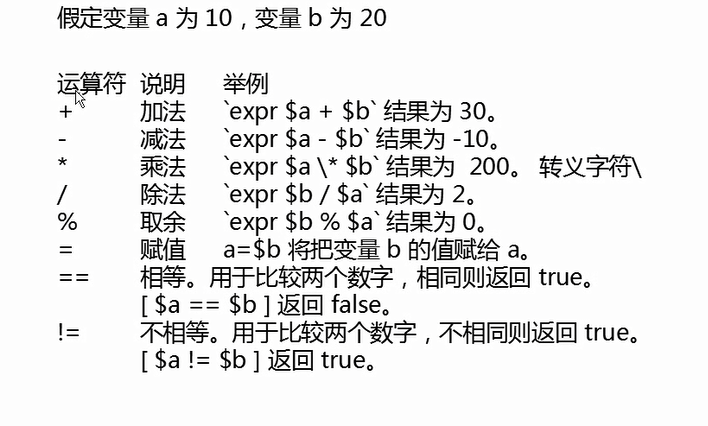假如变量a为7，变量b为3， ①/ 在除法中，则expr$a  /  $b 结果为2 注意：除法中的结果为商 ②% 在取余中，则expr$a  %  $b 结果为1 注意：取余中的结果为余数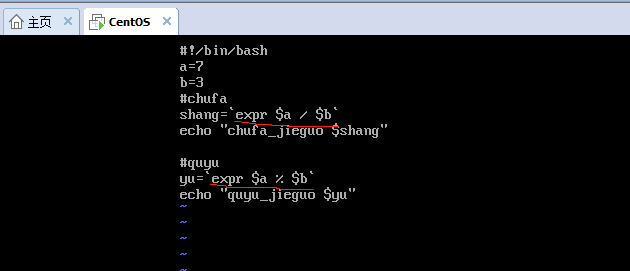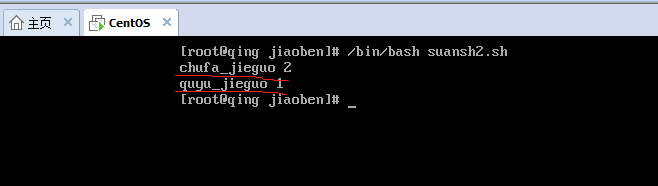③\* 注意： 在乘法中，系统默认*号问一个符号，而不是乘号，所以需要使用转义符号 \ 来帮助系统识别这里是一个乘号 格式 \*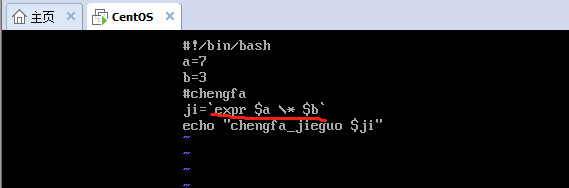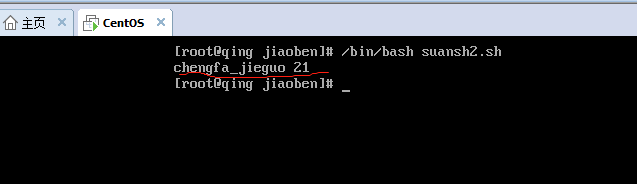④= 这里是赋值符号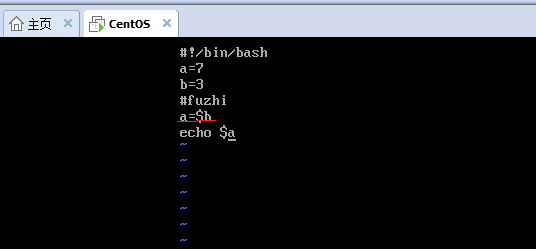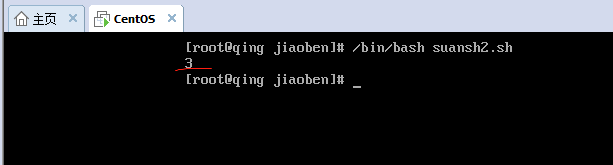⑤== 在相等中，用于比较两个数字，相等则返回true， [$a == $b],不相等则返回false ⑥！= 在不相等中，也是用于比较两个数字，不相等则返回true， [$a  !=  $b],相等则返回false if语句 条件判断语句 语法格式一： if [ 判断条件 ] then 语句块 fi == 判断相等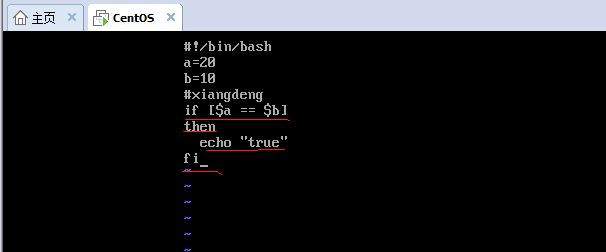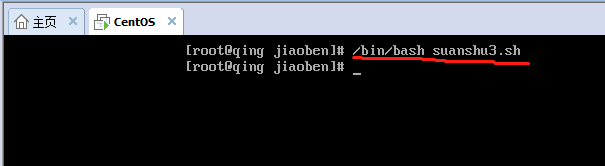！= 判断不相等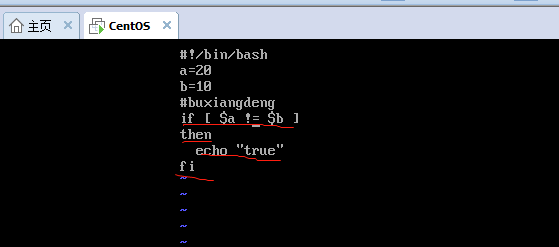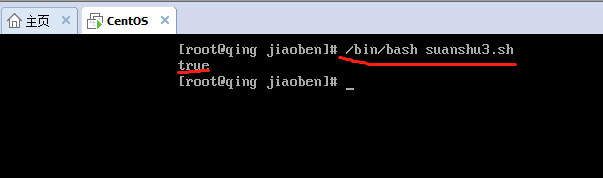语法格式二： if [ 判断条件 ] then 语句块1 else 语句块2 fi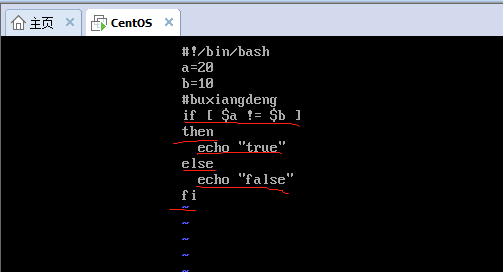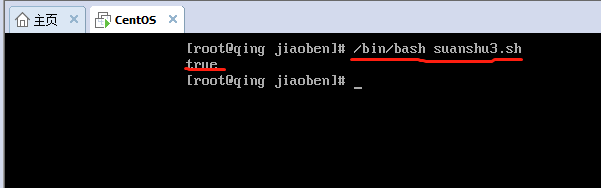二，关系运算符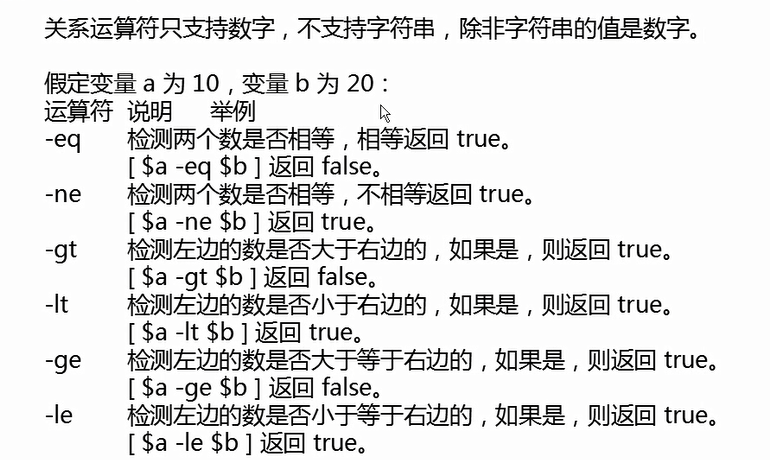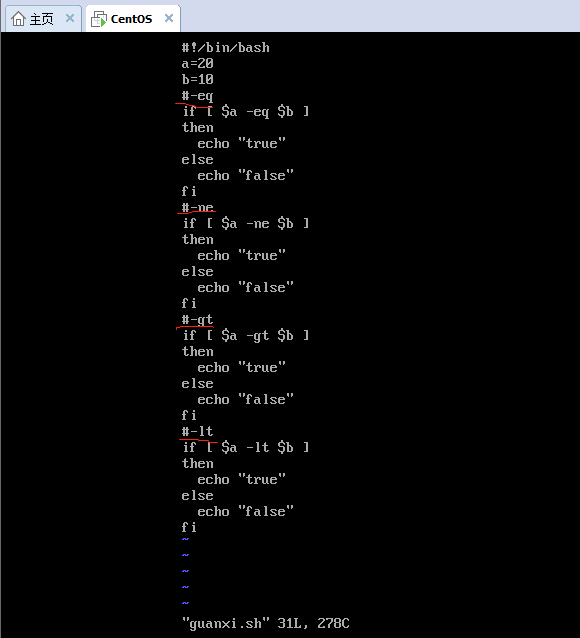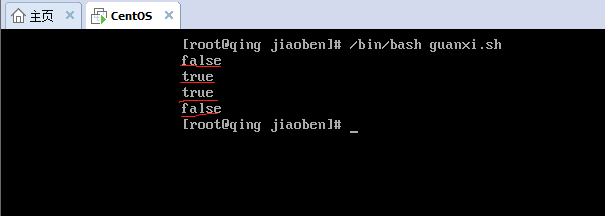三，布尔运算符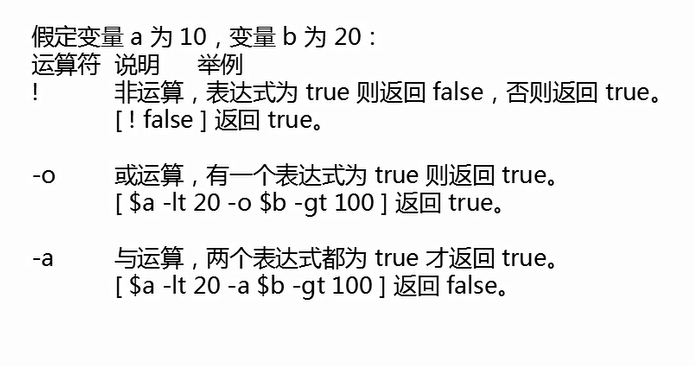①！ 或运算 结果是取反 ②-o 或运算，只要有一个结果为true，最终结果就为true ③-a 与运算，两个结果都是true，最终结果才为true，也可以说，只要有一个结果为false，结果就为false。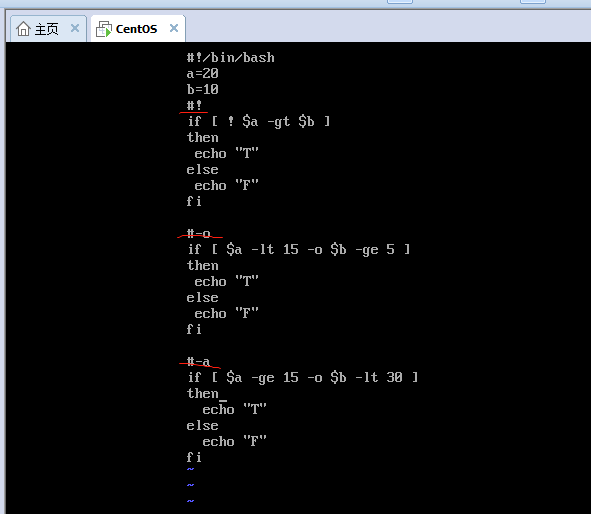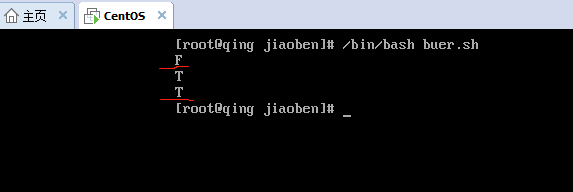四，逻辑运算 假如变量a为100，变量b为200 运算符 说明 举例 && 逻辑与 and 两个结果都为true，最终结果才为true，也就是说，有一个结果为false，最终为false || 逻辑或 or 有一个结果为true，最终结果就为true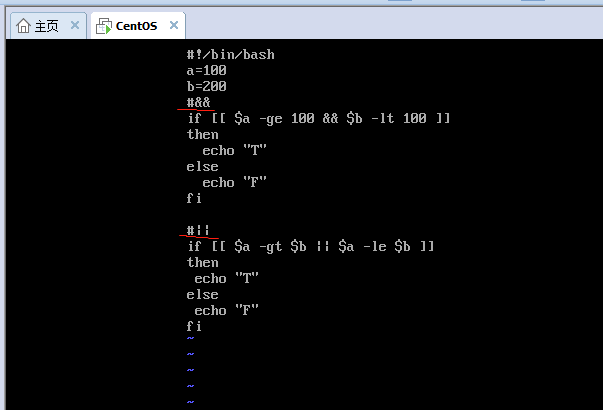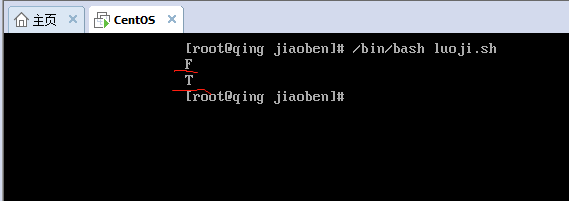五，字符串运算符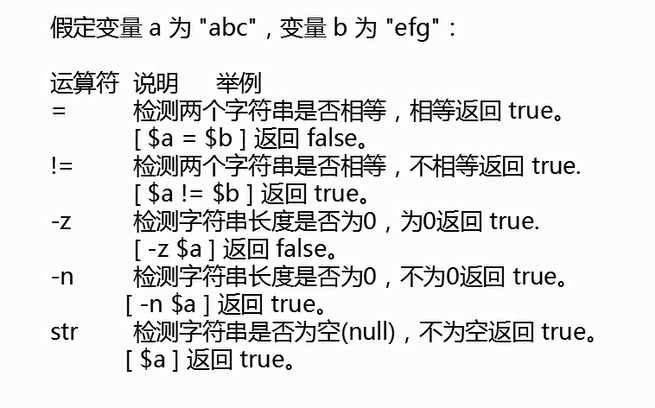注意：最后一个不需要加参数，直接写[$变量名]，字符串不为空返回true。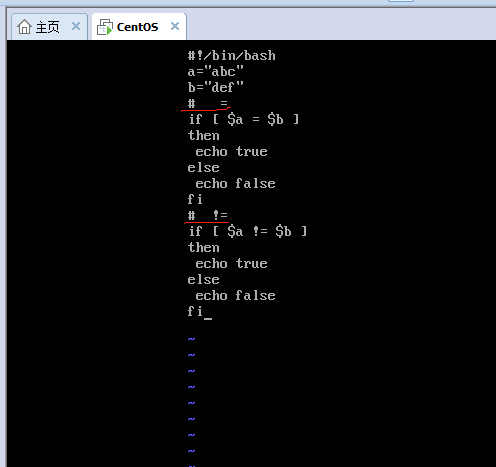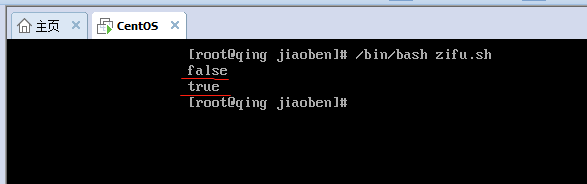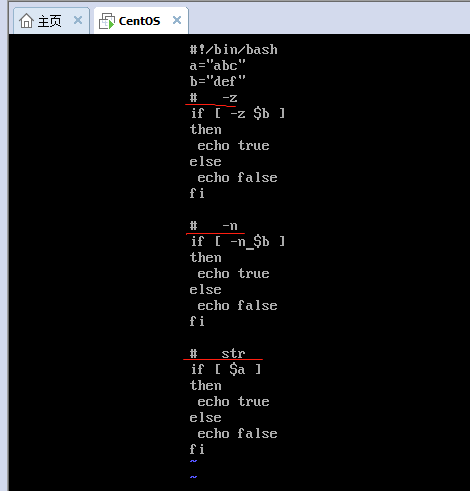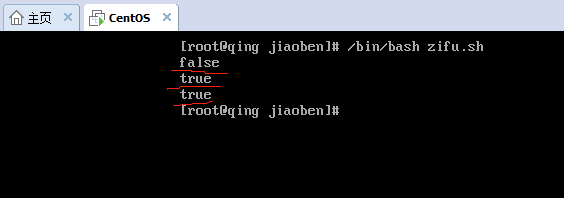六，文件测试运算符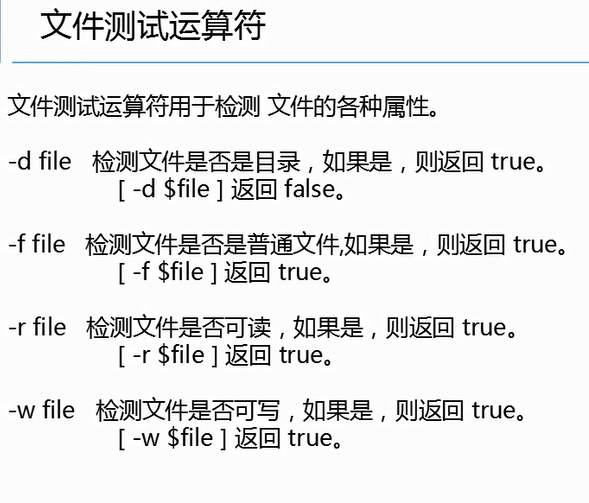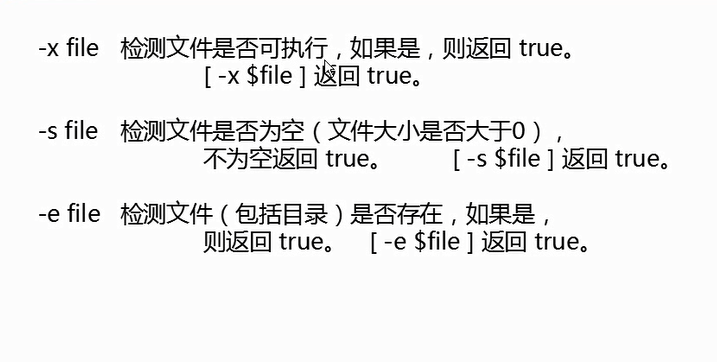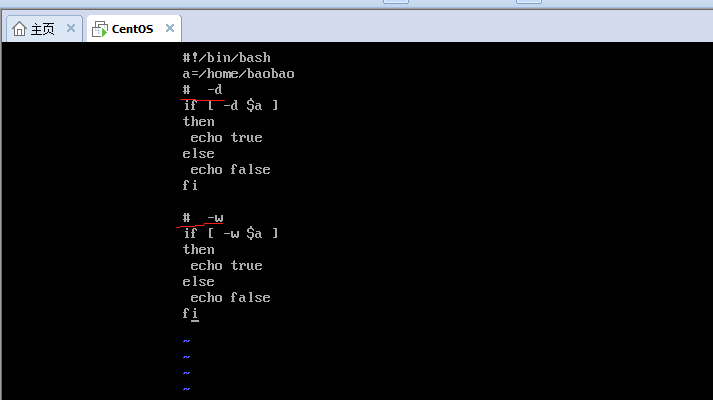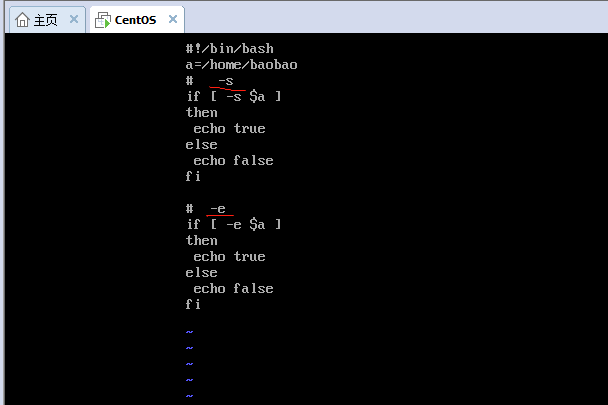展开全文Linux
• 注：此博客使用的是Bash shell 算数运算符 运算符 说明 举例 + 加法 expr $a +$b - 减法 expr $a -$b * 乘法 expr $a \*$b / 除法 expr $b /$a % 取余 expr $b %$a = 赋值 a=$b 将把变量 b...linux • Shell 基本运算符 Shell 和其他编程语言一样，支持多种运算符，包括： 算数运算符 关系运算符 布尔运算符 字符串运算符 文件测试运算符 原生bash不支持简单的数学运算，但是可以通过其他命令来实现，例如 awk 和 ... • Shell 和其他编程语言一样，支持多种运算符，包括： 算数运算符 关系运算符 布尔运算符 字符串运算符 文件运算符 原生bash不支持简单的数学运算，但是可以通过其他命令来实现，例如 awk 和 expr，expr是最常用... • Linux shell 算术运算符 关系运算符 布尔运算符 字符串运算符 文件测试运算符bash • Bash 支持很多运算符，包括算数运算符、关系运算符、布尔运算符、字符串运算符和文件测试运算符。 原生bash不支持简单的数学运算，但是可以通过其他命令来实现，例如 awk 和 expr，expr 最常用。 expr 是一款...linux bash • 1) “$((运算式))”或“$[运算式]，如$((1+2)) $[1+2] 2) expr m + n 注意expr运算符间要有空格 expr 1 + 2 3) expr \*, /, % 乘，除，取余 • (())数学逻辑运算，比较大小 \s:表示是空白符(包括空格，制表，换行，分页等 ) read 可以定一个变量并且从键盘上获取输入的值赋予变量 判断某个变量里面的值不为空时：-n = ! -z 在 [ ]中； 判断数值相等可以用 -eq... • Shell运算符 Shell 和其他编程语音一样，支持包括：算术、关系、布尔、字符串等运 算符。原生 bash 不支持简单的数学运算，但是可以通过其他命令来实现，例如 expr。expr 是一款表达式计算工具，使用它能完成... • Bash 支持很多运算符，包括算数运算符、关系运算符、布尔运算符、字符串运算符和文件测试运算符。 原生bash不支持简单的数学运算，但是可以通过其他命令来实现，例如 awk 和 expr，expr 最常用。 expr 是一款... • 1、shell算术运算符列表： Bash 支持很多运算符，包括算数运算符、关系运算符、布尔运算符、字符串运算符和文件测试运算符。 原生bash不支持简单的数学运算，但是可以通过其他命令来实现，例如 awk 和 expr，expr ...bash • a=10 b=20 echo "a+b = " expr${a} + ${b} echo "a-b = " expr${a} - ${b} echo "a*b = " expr${a} \* ${b} echo "a/b = " expr${a} / ${b} echo "a%b = " expr${a} % ${b}` if [$a == $b ] ... • | 运算符 管道符号，是unix一个很强大的功能,符号为一条竖线:"|"。 用法: command 1 | command 2 他的功能是把第一个命令command 1执行的结果作为command2的输入传给command 2，例如:$ls -s|sort -nr (请注意...
• 傻逼一样的语句，爬爬爬 测试语句 ...垃圾shell,大于小于等于不香吗？ 不能用<>=,我感觉他把这玩意当文件输出了，哎 随便写一个判断文件存在的 存在返回文件名 不存在告辞 ...linux
...